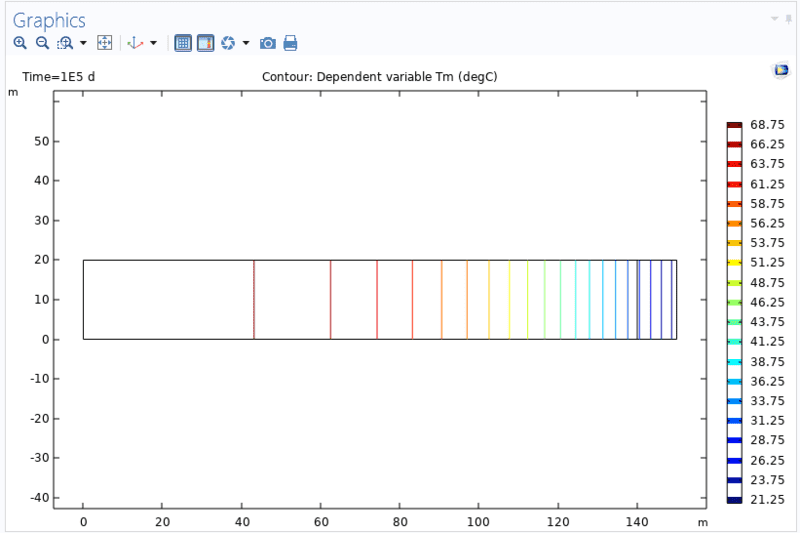# Comsol: Infinite Element Domain module

Hello folks,
I want to simulate a 2D heat transfer process in the subsurface on a region which is infinite on the r-direction. So, as you know, the very basic way to model this is to draw a geometry that is very long in the r direction. I have done this, and the results that I obtain is correct as in this case, the results are matched with the analytical solution. As you know, there is a capability in Comsol called infinite element domain which serves the purpose to the problem mentioned above. In this case, we need to define a limited geometry on which we want to solve the PDE, and also need to draw a small domain acting as the Infinite Element Domain. However, in this case, the results are not correct because they are not matched with the analytical solution. Is there anything that I am missing to correctly use Infinite Element Domain in Comsol?
Any help or comment would be appreciated.

There are some examples of analyses involving infinite elements in Comsol's Application Gallery. Have you checked them ?

•There are some examples of analyses involving infinite elements in Comsol's Application Gallery. Have you checked them ?
Yup, I have checked them, and I think I am doing it right for the problem that I am considering. I have a question; to numerically solve an infinite region problem, one way is to draw a very lengthy region, right? And, now one functionality of infinite elements domain feature of Comsol is to replace this infinite region with a finite region and then stick to it an infinite element domain, right?

Yes, with sufficiently large models it’s usually possible to approximate unbounded (or almost unbounded when compared with the ROI) domains. But infinite elements allow you to reduce the computational effort by performing the analyses on much smaller geometries. Those elements are often used in acoustics and even solid mechanics (e.g. infinite plates).

Can you attach some pictures showing your model ? It would be easier to give some advice.

•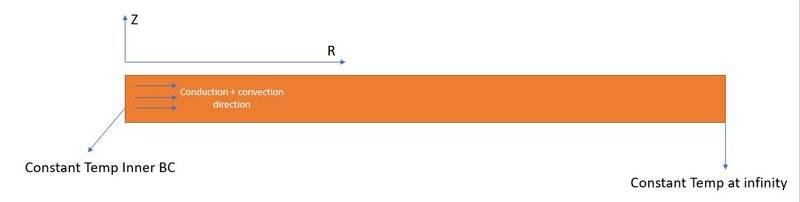At the inner BC, a fluid at constant temp is injected, and far at the infinity, the boundary condition is another constant temperature (lower than that at the inner BC). Also, the temp at initial condition is the same as that at the outer boundary condition. Now, the general way to model this is to draw this geometry whose length in the r direction is large. The simulation results are correct until the temperature at the outer boundary condition starts to increase. The problem has an analytical solution, based on which I have verified the numerical results.

Another way to model this, is to use infinite element domain in Comsol. For this, the above geometry can be replaced with: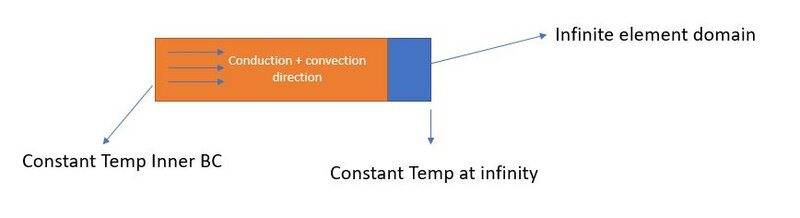In this case, I assume even if temp at the outer boundary (at the bounded region, not at the infinite element domain) starts to increase, the results should be correct. However, the results in this case, is incorrect (unverified against the analytical solution). I wonder what I am missing, or if I am doing something wrong in Comsol?

Thanks again.

Last edited:
What are the differences in the results (analytical, finite element only model and the one with infinite elements) ?

•Here are the results that I get: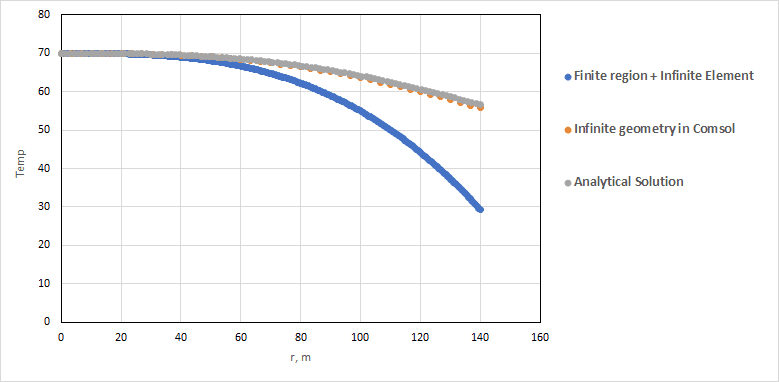Either I am missing something on how I should implement this Infinite Element in Comsol, or I am mistaken to believe that Infinite element works for this scenario.

Let me elaborate more on the problem:
A fluid with high temperature is being injected into a region with lower temperature:The equation to solve is: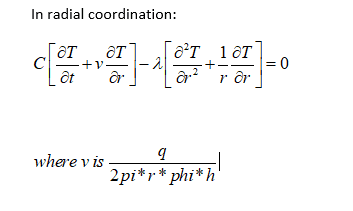With the following initial and boundary conditions (note that the upper and lower boundary condition is no-flux):We want to obtain the temperature profile over the length of rw<r<140 m (rw is very small and is equal to 0.005 m here) at different times. One way to model this numerically in Comsol is to draw a rectangle that is 2000 m in the r-direction, and get results only in the span of r [rw,140] m: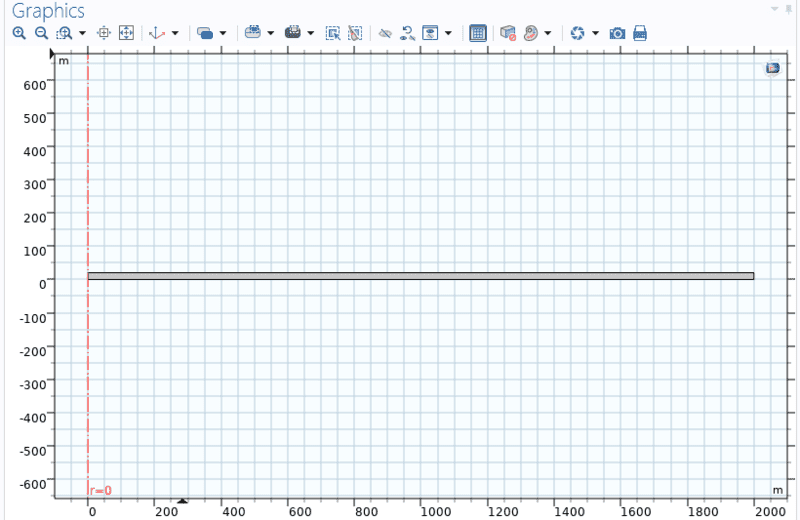The results of this case is fine, because they are well-matched with the analytical solution.

Another way to model this is to replace the above geometry with a bounded one that is [rw, 140] m in the r-direction and then augment it with an Infinite Element domain that is meshed mapped, as follows: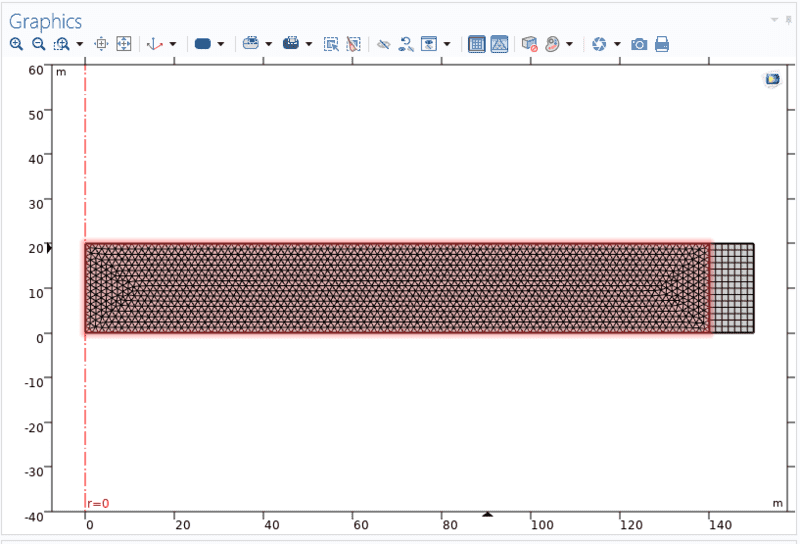Here, I have set the thickness of Infinite Element to 10 m in the r-direction. However, the results in this case are not matched with the analytical solution (or the above case where Infinite Element domain was not used). Is there anything that I am missing in Comsol? I have also changed some variables with regard to Infinite Element in Comsol such as physical width or distance, but I didn't see any changes in the results.

Do the temperature and heat flux contour plots look correct in the case when infinite elements are used ? Are their distributions continuous at the interface of these two domains ?

The temperature profile: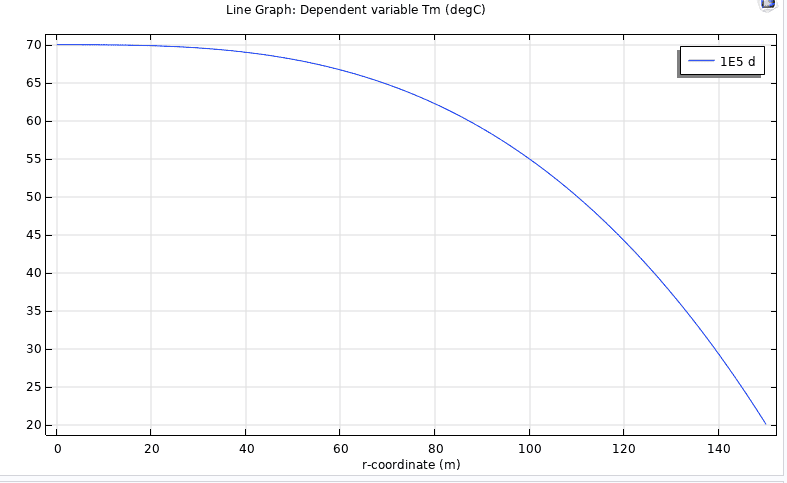The temperature gradient (representing heat flux) profile: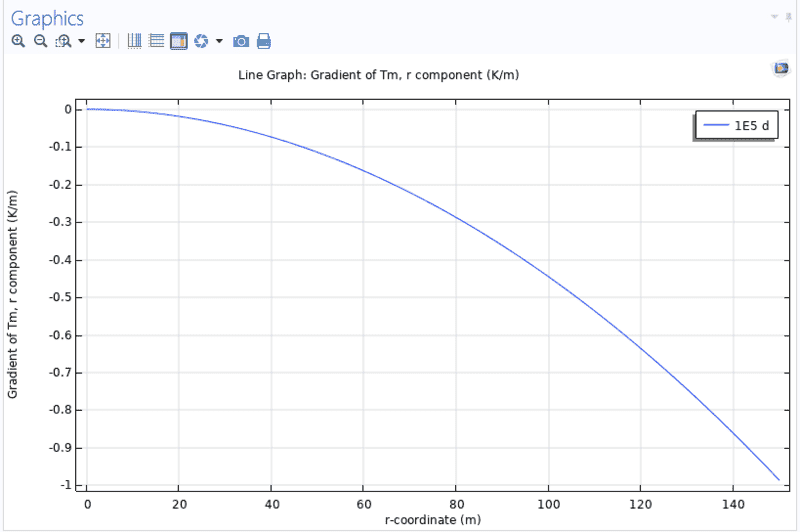The bounded region or the so-called ROI is 0.005<r<140 m and physical width of infinite element in the r-direction is 10 m. As you can see, the temperature and its gradient are both continuous at the interface (r=140 m).

As far as temperature and flux contours in both the bounded region and infinite element are concerned, I don't see anything suspicious. Here, for example, the contour map of temperature is: